# Python Program to Multiply Two Matrices

In this tutorial you will learn about the Python Program to Multiply Two Matrices and its application with practical example.

In this tutorial, we will learn to create a Python Program to Multiply Two Matrices using python Programming.

## Prerequisites

Before starting with this tutorial we assume that you are best aware of the following Python programming topics:

• Python Operator.
• Basic Input and Output.
• Basic Python  programming.
• Python Data type.
• Python in-built Functions.
• Loops in Python.
• List in Python.

## Matrix Multiplication ?

A Matrix is a table consist of numbers.Let take an example how of matrix multiplication takes place.If we want to multiply 2 in our 2*2 matrix then how it will be done as shown in image below.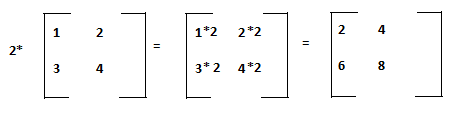As we cane see in the image above that multiplying a matrix by a single number (2) is shown in image above Means 2 is multiply with each and every element.

 2×1=2 2×2=4 2×3=6 2×4=8

## Python Program to Multiply Two Matrices

In this program below we create a program to multiply two Matrices using nested for loop . We would first declared and initialized the required variables. Next, we would prompt user to input the values and later we will multiply two matrices.

## Output

Matrix A and B: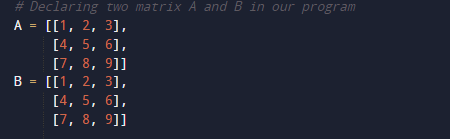Result: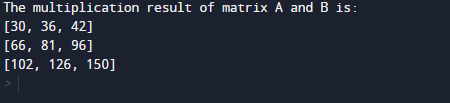In our program above  we going to use nested “for” loops to iterate through each and every row’s and column’s. within the loop we “Multiply” the corresponding elements of the two matrices and store it in variable “result”.

In the above program, we have first declared and initialized a set variables required in the program.

• A = it will hold first matrix.
• B= it will hold second matrix.
• result=it will hold added values of matrices.
• i,j and k = For iteration.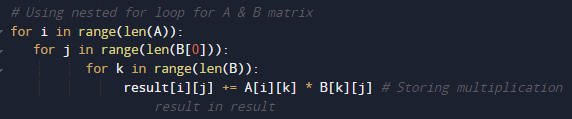Here we will multiply two matrices of 3*3  using nested for loops to iterate through each row and column. We multiply  the corresponding elements values of  two matrices and store it in the result variable.At last we will display the value stored in variable “Result” after Multiplying of two matrices .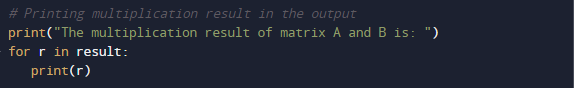In matrix multiplication of two matrices, the first row elements of matrix(A) are multiplied to the column elements of the second matrix (B). And this process followed till we reach all the element of rows.And finally we get resultant Output we required.

In this tutorial we have learn about the Python Program to Multiply Two Matrices and its application with practical example. I hope you will like this tutorial.